Module 5 - Limits and Infinity Introduction | Lesson 1 | Lesson 2 | Lesson 3 | Lesson 4 | Self-Test Lesson 5.4: Nonlinear Asymptotes In this lesson you will examine a rational fraction with a nonlinear asymptote. Using propFrac( will be helpful in determining the equation of the nonlinear asymptote. 5.4.1 Use your TI-89 to rewrite the rational function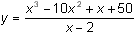as a polynomial plus a proper fraction. Click here for the answer. 5.4.2 What do the parts of the proper fraction tell you about the graph of? Click here for the answer. Provide graphical support for the answer to Question 5.4.2 by graphing the function in a large window and in a window near x = 2. The Wide View Enterinto y1 and y = x2 – 8x – 15 into y2. Set the Window values to [-50, 50] x [-2500, 2500] with xscl = 10 and yscl = 500.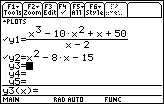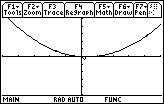The graph oflooks like the graph of the parabola y = x2 – 8x – 15 in this large viewing window. The Narrow View Replace y2 with the function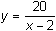Set the Window values to [0, 4] x [-500, 500] with xscl = 1 and yscl = 50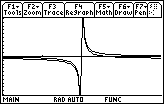The graph oflooks like the graph ofin this window. A Medium View To show both the vertical and parabolic asymptotic behavior ofDelete y2 in the Y= Editor Graph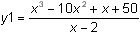with line style Graph y2 = x2 – 8x – 15 with dot style. First enter y2 = x^2 - 8x - 15. Then place the cursor anywhere in y2 and press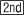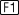. Finally press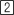.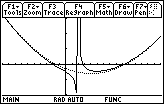Set the Window values to [-10, 15] x [-100, 150], xscl = 1, yscl = 50 As you have seen, the choice of viewing window can dramatically affect the appearance of a function, and different windows should be used to illustrate different features. The best graph is one in which all significant features of a function are displayed or implied. Such a graph is called a complete graph. Two or more windows are often needed to illustrate a complete graph. < Back | Next > ©Copyright 2007 All rights reserved. | Trademarks | Privacy Policy | Link Policy